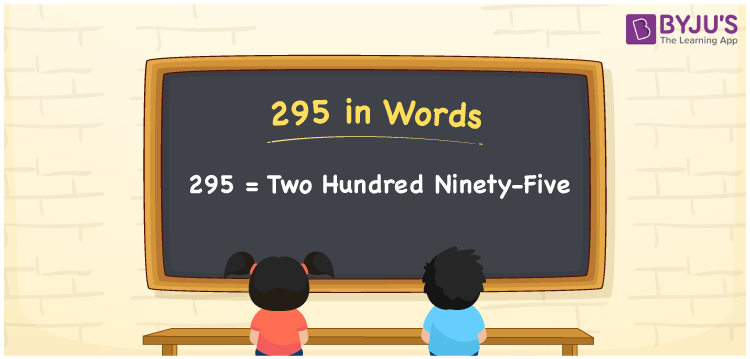# 295 in Words

The number 295 can be represented as Two Hundred Ninety-Five. For example, if you purchased a headphone worth Rs. 295, then you can say, “I purchased a headphone worth Rupees Two Hundred Ninety-Five”. 295 is a cardinal number as it denotes a specific amount. Let us have a look at how the conversion of numbers to words is easy with the help of a place value table in this article. Also, learn the spelling of the number 295 in English.

 295 in Words Two Hundred Ninety-Five Two Hundred Ninety-Five in numerical form 295

## 295 in English Words

Students are taught to write the numbers in words with the help of the English alphabet since it is a widely used language in education. Therefore, we can write the number 295 in words as Two Hundred Ninety-Five.## How to Write 295 in Words?

295 is a three-digit number. Hence, we need to make a chart of 3 columns to represent its place value chart. The place value chart for the number 295 is as follows.

 Hundreds Tens Ones 2 9 5

Hence, we can write the expanded form as:

2 x Hundred + 9 x Ten + 5 x One

= 2 x 100 + 9 x 10 + 5 x 1

= 200 + 90 + 5

= 295

= Two Hundred Ninety-Five

Hence, 295 in words is written as Two Hundred Ninety-Five

Interesting way of writing 295 in words

2 = Two

29 = Twenty-Nine

295 = Two Hundred Ninety-Five

Thus, the word form of the number 295 is Two Hundred Ninety-Five

295 is a natural number that is one less than 296 and one greater than 294.

• 295 in words – Two Hundred Ninety-Five
• Is 295 an odd number? – Yes
• Is 295 an even number? – No
• Is 295 a perfect square number? – No
• Is 295 a perfect cube number? – No
• Is 295 a prime number? – No
• Is 295 a composite number? – Yes

## Frequently Asked Questions on 295 in Words

Q1

### How do you write 295 in words?

295 in words is written as Two Hundred Ninety-Five.
Q2

### Simplify 200 + 95, and express in words.

Simplifying 200 + 95, we get 295. Thus, the number 295 in words is Two Hundred Ninety-Five.
Q3

### 295 is a prime number. True or False.

False, the number 295 is not a prime number.